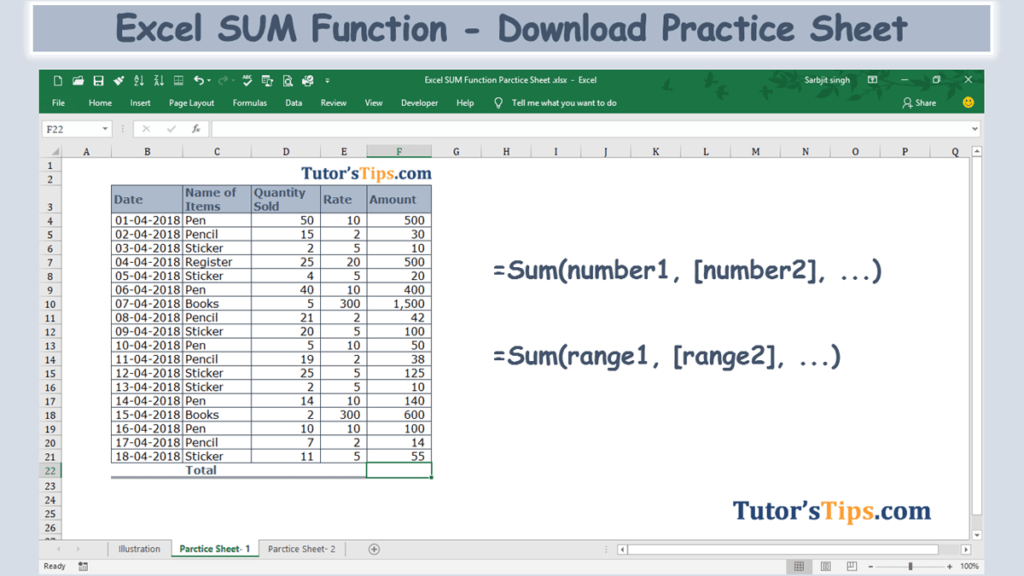The Excel SUM Formula is used, where we need to do the sum of the numerical values in the selected range, cells reference or table column/row. It can sum together the numerical values in the multiple selected ranges or cells references.

## The feature of “SUM” function: –

• With the help of the SUM Function, we can get the total of the numerical values in the selected array, range or cells.
• It can use further with other excel function.
• Error values or text values that cannot be recognized into numbers are not summed by this function.
• The logical values TRUE and FALSE are also not summed.
• Empty cells and text are also not summed.

### Explanation of Function:-

Now, We will explain the Arguments of the Function.

=SUM(number1, [number2], [number3], …)

number1: – Number 1 is represented as the first selected cell which we want to sum up.

[number2]: – Number 2 is represented the next selected cell which we want to sum up.

[number3]: – Number 3 is represented the next selected cell which we want to sum up and a process of selection going on.

We can also select range instead of selecting a number individually.

=SUM(range1, [range2], [range3], …)

range1: – Range 1 is represented as the first selected cells which we want to sum up.

[range2]: – Range 2 is represented the next selected cells which we want to sum up.

[range3]: – Range 3 is represented the next selected cells which we want to sum up and a process of selection going on.

### Example of Function: –

I will show you, how to apply this function.

From the following table get the total of the amount.

Solution:-

We will Apply the SUM Formula and get the result. This is shown in the following steps: –

Step No. 1:-

We will write the “=SUM( ” in the column of the result.

Step No. 2

Now select the range of cells on which you want to apply the function.

Step No. 3: –

Now, Press enter and get the result: –

Thanks